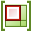# Creating the Model

Create the model in CADFEKO. Define any ports and sources required for the model. Specify the operating frequency or frequency range for the model.

1. Set the model unit to millimetres.
2. Define the following variables:
• freq = 10e9 (The operating frequency.)
• lambda = c0/freq*1e3 ( The wavelength in free space. Note the unit is in millimetres.)
• n = 1 (Feed pin position index.)
• pin_step_size = lambda/32 (Distance between the pin positions.)
• pin_length = 0.9*lambda/4 (Length of pin feed monopole.)
• pin_offset = pin_step_size*n (Pin offset from waveguide tip.)
• waveguide_length = lambda*2 (Length of waveguide section.)
• wr90_height = 10.16 (Waveguide height for WR90 (X-Band, 8.2-12.4 GHz).)
• wr90_width = 22.86 (Waveguide width for WR90 (X-Band, 8.2-12.4 GHz).)
Note: The model unit is millimetres. The variable lambda is not the actual wavelength but a parameter used in the geometry definition.
3. Create the waveguide.
1. Create a cuboid.
• Definition method: Base centre, width, depth, height
• Base centre: (0, 0, 0)
• Width: wr90_width
• Depth: waveguide_length
• Height: wr90_height
• Label: waveguide
4. Set the waveguide region to Free space.
5. Imprint vertices on the mesh for the feed pin connections.
1. Create a line.
• Start point: (0, -waveguide_length/2, 0)
• End point: (0, -waveguide_length/2 + pin_step_size, 0)
• Label: imprinted_edge_1
2. Copy and translate imprinted_edge_1.
• From: (0, 0, 0)
• To: (0, 2*pin_step_size, 0)
• Number of copies: 7
6. Union all geometry. Rename the resulting part to waveguide_perforated.
7. Activate the NGF and set waveguide_perforated as a static part.
Note: Theicon in the model tree, next to waveguide_perforated, indicates it is a static part and may not be edited.
8. Create the feed pin.
1. Create a line.
• Start point: (0, pin_offset, 0)
• End point: (0, pin_offset, pin_length)
• Workplane origin: (0, -waveguide_length/2, 0)
9. Add a wire port at the connection point between the feed pin and the waveguide floor.
10. Place a waveguide port at the opposite waveguide end. This will absorb the power injected by the pin feed.
1. Rotate the reference direction with 90°.
11. Add a voltage source to the wire port. (1 V, 0°, 50 Ω).
12. Set the frequency to freq.
Note: A magnetic plane of symmetry exists about the X=0 plane, but no computational performance benefit is obtained when used in conjunction with the NGF.